# IMU¶

IMU include MoonBot Kit Controller Module three-axis acceleration , drive of three-axis magnetometer and temperature sensor on board.

By calling these modules, you can get MoonBot Kit master control of current direction, tilt angle and state, etc.## Compass calibration¶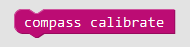Introduction

When calibrating the compass, the master control needs to flip in the shape of ”∞“

## Acquisition of compass angle¶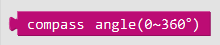Introduction

Read the angle between the current direction and the northward direction of the compass Y axis.

Return
• `0~360°`

## Acquisition of acceleration value¶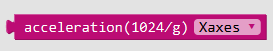Introduction

Read the acceleration simulation of a given axis.

Parameters
Directional axis
• `X,Y,Z`

Return
• `Acceleration analogue`

## Acquisition of acceleration angle¶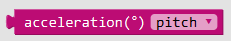Introduction

Acquisition the tilt angle of the master control

Introduction
Angle type
• `Elevation angle` :The angle between Y axis and horizontal plane in the master control coordinates. When the main control tilts upward, the pitch angle is positive and vice versa.

• `Roll angle` :The angle between X axis and horizontal plane in the master control coordinates. When the main control tilts to the right, the roll angle is positive and vice versa.

Return
• `±180°`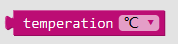Introduction

Parameters
Unit of temperature
• `℃` :Celsius degree

• `℉` :Fahrenheit degree

Return
• `Temperature value`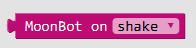Introduction

Read the current master control state.

Parameters
state
• `shock` :Whether the master control is in vibration state or not

• `Free fall` :Whether the master control is in free falling state

Return
• `true` :The master control is currently in this state

• `false` :The master control is not currently in this state